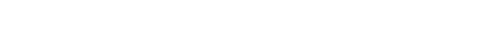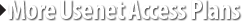# Binary Converter

 Quantity to be converted: From: Bit Byte Kilobyte (KB)  Megabyte (MB) Gigabyte (GB) Terabyte Petabyte To: Bit Byte Kilobyte (KB)  Megabyte (MB) Gigabyte (GB) Terabyte Petabyte

Most of us might not know what is the difference between kilobyte and kibibyte. One of the most confusing problems regarding PC statistics and measurements, where people misuse kibibytes as kilobytes because, the computers are more widely used nowadays, so the binary nature of digital computer lends them to think kilobyte as 1024. 1024 bytes is not really a kilobyte, it is a kibibyte. Kilo stands for 10^3, which is 1000, which is not equal to 1024. Kilobyte is a unit of information equal to 1000 bytes in SI unit. As a unit of computer storage, i.e., in Binary, the kilobyte is usually equal to 1024 bytes, this should be called as kibibyte. Don't get confused with kilobyte and kibibyte which lets you to calculate wrongly. The following table will help you in this difference.

 Name(SI) Symbol(SI) SI Value Name(Binary) Symbol(Binary) Binary Value kilobyte KB 103 = 1000 Bytes kibibyte KiB 210 = 1024 Bytes megabyte MB 106 = 10002 Bytes mebibyte MiB 220 = 10242 Bytes gigabyte GB 109 = 10003 Bytes gigabyte GiB 230 = 10243 Bytes kilobit kb 103 = 1000 Bits kibibit Kibit 210 = 1024 Bits megabit Mb 106 = 10002 Bits Mebibit Mibit 220 = 10242 Bits gigabit Gb 109 = 10003 Bits Gibibit Gibit 230 = 10243 Bits

The smallest unit of information in a system. A bit has a single binary value, either 0 or 1. A four-bit quantity is known as a nibble.

Byte : A Byte is a series of 8 bits. It is also called as character. Computer storage space is usually measured in Bytes. It is a sequence of bits processed as one unit of information.Comprised of eight bits, they are large enough to hold one character of alphanumeric data.

Kilobyte : Kibibyte
A kilobyte or KB is approximately 1000 bytes(103) in SI value. Kibibyte is a unit of information or computer storage, which are used to measure memory capacity.A kibibyte or KiB is approximately 1024 bytes(210) in Binary value.

Megabyte : Mebibyte
A megabyte or MB is approximately 1000000 bytes(106) in SI value. Mebibyte is an amount of computer memory consisting of about one million bytes. A mebibyte or MiB is approximately 1,048,576 bytes(220) in Binary value. Gigabyte : Gibibyte
A gigabyte or GB is approximately 1000000000 bytes(109) in SI value. Gibibyte is a measure of computer data storage capacity about one billion bytes. A gibibyte or GiB is approximately 1,073,741,824 bytes(230) in Binary value.

Kilobit : Kibibit
A kilobit or kb is approximately 1000 bits(103) in SI value. Kibibit is a data transmission speed measure. A kibibit or Kibit is approximately 1024 bits(210) in Binary value.

Megabit : Mebibit
A megabit or Mb is approximately 1000000 bits(106) in SI value. Mebibit is used to measure higher rates of data transfer, it consist of one million bits. A mebibit or Mibit is approximately 1,048,576 bits(220) in Binary value.

Gigabit : Gibibit
A gigabit or Gb is approximately 1000000000 bits(109) in SI value. Gibibit is used for measuring the amount of data that is transferred in a second between two telecommunication points, it consist of one billion bytes. A gibibit or Gibit is approximately 1,073,741,824 bits(230) in Binary value.